# Chapter 2

### Chapter 2 Code Examples

#### MATLAB

x = 2
five = 5
z = 3.14159
my_string = ‘Hello World!’
traffic=[200,150,350,235,450];
nox = zeros(1,365);
x=[1, 2, 3, 4];
y=[1, 2; 3, 4];
x=zeros(6)
y=ones(8)

helloworld.m

% Hello world

'Hello World'
disp 'My name is "Hal".'

#### Python

x = 2
five = 5
z = 3.14159
my_string = ‘Hello World!’
raffic=[200,150,350,235,450]
nox = [0.]*365
import numpy as np
x=np.array([1, 2, 3, 4])
y= np.array([[1, 2], [3, 4]])
x=np.zeros(6)
y=np.ones(8)

temp.py

"""
Spyder Editor

This is a temporary script file
"""
print("Hello World")

## Exercises

### 2.3.1 Basic Arithmetic

Convert the following equations into valid MATLAB or Python commands, and submit both the input and output of the command from the interpreter.

1.2.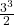3.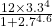### 2.3.2 Creating & Editing Matrices

Create the following matrices, and submit both the input and output of the command from the interpreter.

1.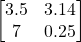2.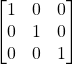3.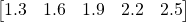For the following problems, create the matrices as described, and then edit as directed, and submit the editing command and the output from the interpreter.

1. Create the array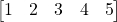, and replace the third element with the number 9.
2. Create the array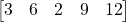, and replace the second element with the value in that location cubed. That is, cube the value found in that location and store it back in the same location.
3. Create the identity matrix and replace the element at location 2,3 with the number of elements in the identity matrix.

Use the materials on the book website to download the data set necessary for these problems in either MATLAB or Python.

1. Load the chapter 2 data set from the zip file below (Chapter2.m or Chapter2.py). What is the value in variable “x”?
2. Clean out the variables in your Workspace or Variable Explorer. Load the chapter 2 data set. Modify the variable “z” to equal . Save the dataset, and submit.
% Script for debugging exercises in Chapter 2

disp 'Chapter 2 Debugging script'

% Set initial variable values

z=0;

% Is this the student version of MATLAB?

if (isstudent)
disp 'This is the student version of MATLAB';
else
disp 'This is not the student version of MATLAB';
end

disp 'Starting loop...'

for x = 0:.2:pi
y=sin(x);
z=z+y;
end

disp 'Loop complete'

clear x y z

disp 'Exiting program.'
# -*- coding: utf-8 -*-
"""
Script for debugging exercises in chapter 2

@author: guilfoos
"""
import numpy as np
import math

print("Chapter 2 Debugging script")

# Set initial variable values
z=0

print("Starting loop...")

for x in np.arange(0,math.pi,.2):
y = math.sin(x)
z = z + y

print("Loop complete")

x=None
y=None
z=None
del x
del y
del z

print("Exiting program.")